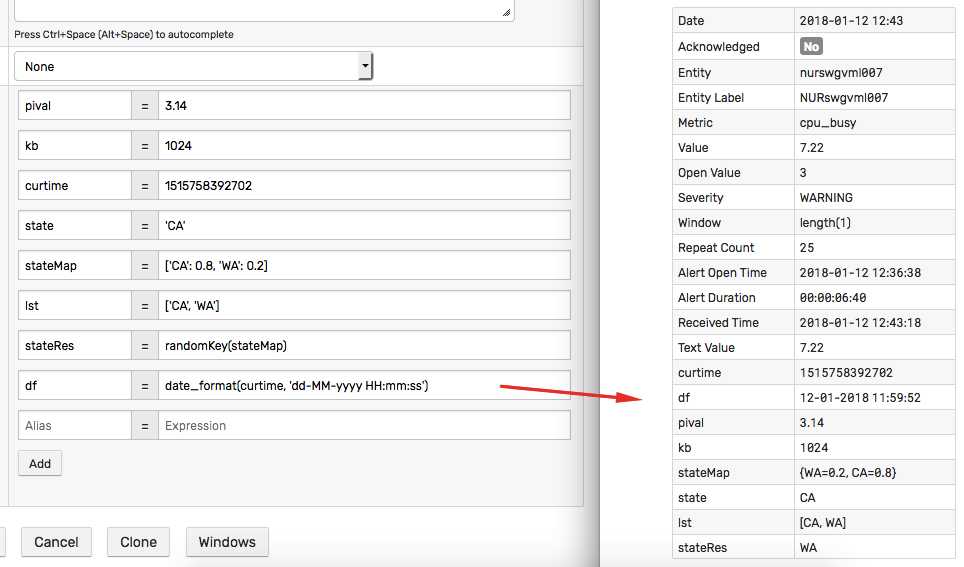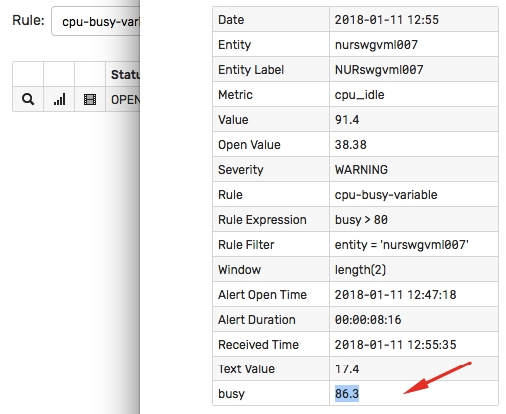# # Variables

## # Overview

Variables are custom fields defined by the rule author on the Condition tab. Each variable consists of a unique name and an expression.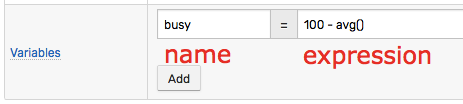## # Usage

### # Condition

The user-defined variables can be referenced in the rule condition.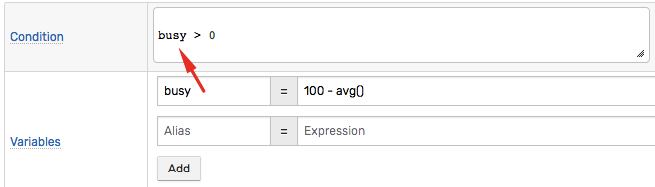### # Response Actions

Similar to the standard window fields, variables can be included by name in the notifications messages, scripts, and logging messages using placeholders:

``````\${busy}
``````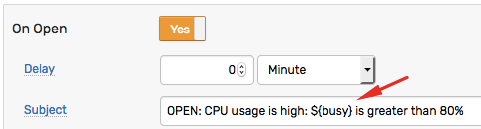### # Filter

Variables cannot be included in a filter expression because filters are evaluated prior to the command being added to a window.

## # Data Types

### # double

``````pival = 3.14
``````

### # integer

``````kb = 1024
``````

### # long

``````curtime = 1515758392702
``````

Big Integers

Define large integers as Long (64-bit integer) or floating numbers to avoid 32-bit integer overflow. The range of values that a 32-bit integer can hold is limited to `[-2147483647, 2147483648]`.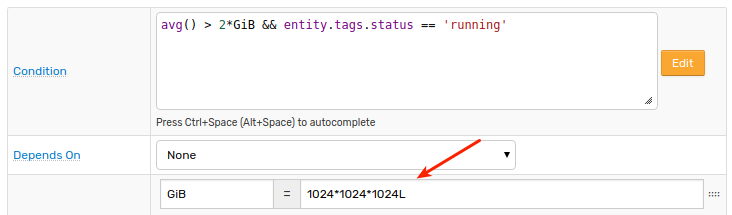### # string

Use single or double quotes when declaring a string variable.

``````state = 'CA'
``````

Inner quotes can be escaped with backslash.

``````sqlQuery = 'SELECT value AS used_mb FROM "fs.rw" WHERE entity = \'' + entity + '\''
``````
``````sqlQuery = "SELECT value AS used_mb FROM \"fs.rw\" WHERE entity = '" + entity + "'"
``````

### # collection

``````errorCodes = [401, 403, 404]
``````

A collection can include elements of different types.

Both single and double quotes can be used to specify elements of string type.

``````stateList = ['CA', 'WA']
``````
``````stateList = ["CA", "WA"]
``````

To check the size of the collection, use the `.size()` method.

To access the n-th element in the collection, use square brackets `[index]` or the `get(index)` method (starting with `0` for the first element).

``````// define variable 'authors'
authors = split(tags.authors, ',')
// define variable 'author'
author  = authors.size() == 0 ? 'n/a' : authors
``````

### # map

``````stateMap = ['CA': 0.8, 'WA': 0.2]
``````
``````countryMap = ['USA': 'North America', 'Brazil': 'South America']
``````

Use single quotes to enclose keys and values.

### # function

``````last_msg = db_message_last('1 week', 'alert', 'rule-engine')
``````
``````since_start = formatIntervalShort(elapsedTime(property('dkr.state::started')))
``````
``````server = upper(keepBefore(entity, ':'))
``````

### # expression

``````annotation = tags.note == null ? 'N/A' : tags.note
``````

Variables can be included in the condition expression.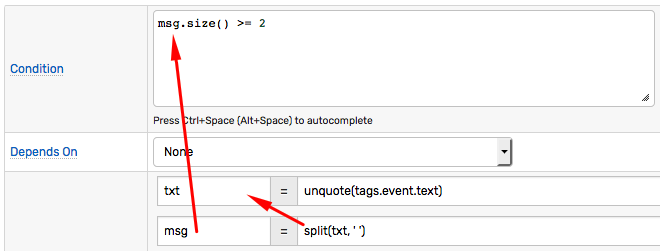The variables can refer to other variables declared in the same rule.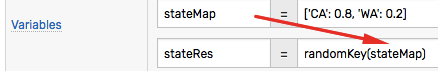The order of variables is important. The dependent variables must be declared after the referenced variables.

``````// Incorrect order
b = a * 2
a = 10
``````

Variable `b` refers to variable `a` and therefore must be declared after `a`.

``````// Correct order
a = 10
b = a * 2
``````

## # Execution

Depending on the sequence of boolean checks in the condition, referenced variables can be evaluated for each incoming or exiting command.

If the window status is `OPEN` or `REPEAT`, all variables are evaluated regardless of condition.

If a variable invokes an external function such as `scriptOut`, such function must execute quickly, within a few seconds.

WARNING

Avoid calling long-running functions in variables.

The current value of a variable can be accessed on the Window Details page.